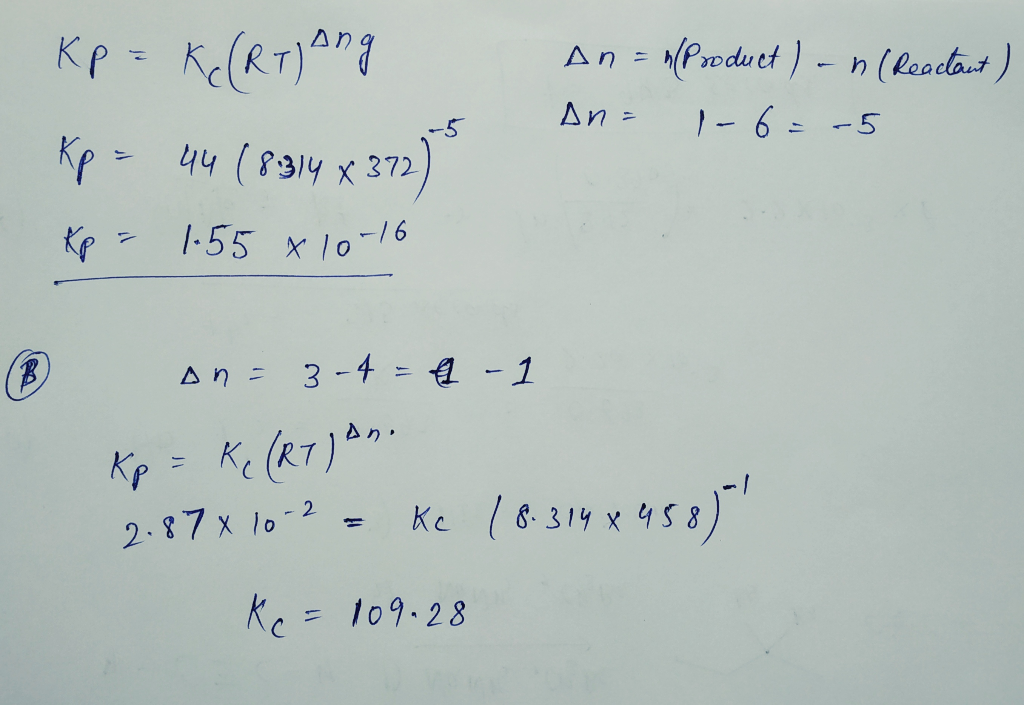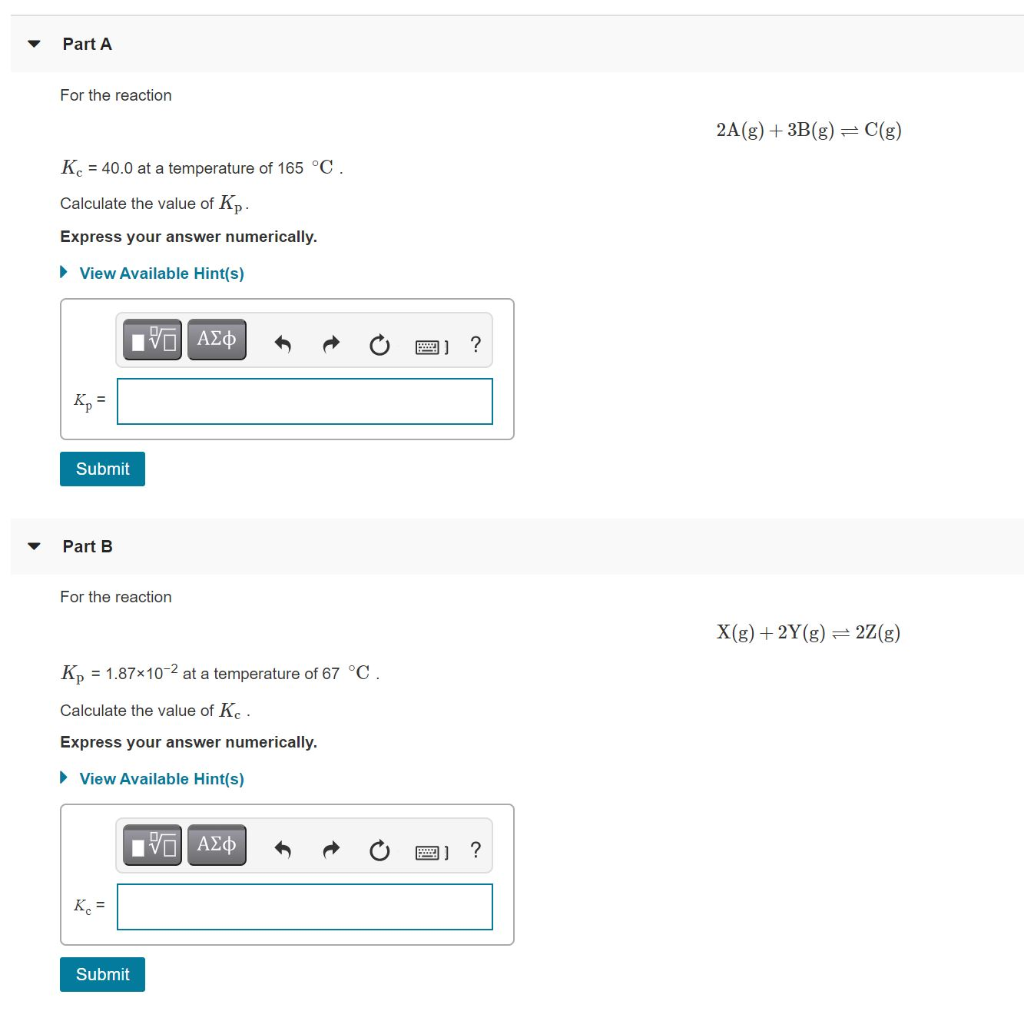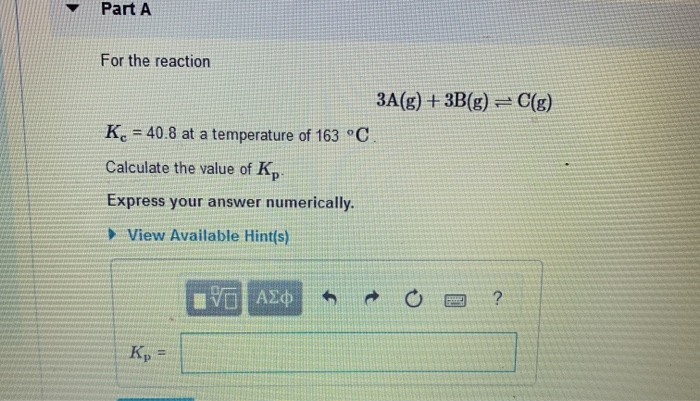Question

# A. For the reaction 3A(g)+3B(g)⇌C(g) Kc = 44.0 at a temperature of 99 C . Calculate...

A. For the reaction

3A(g)+3B(g)⇌C(g)

Kc = 44.0 at a temperature of 99 C .

Calculate the value of Kp.

B. For the reaction

X(g)+3Y(g)⇌3Z(g)

Kp = 2.87×10−2 at a temperature of 185 ∘C .

Calculate the value of Kc .#### Earn Coins

Coins can be redeemed for fabulous gifts.

Similar Homework Help Questions
• ### Part A For the reaction 3A(g)+3B(g)⇌C(g) Kc = 35.0 at a temperature of 347 ∘C ....

Part A For the reaction 3A(g)+3B(g)⇌C(g) Kc = 35.0 at a temperature of 347 ∘C . Calculate the value of Kp. Express your answer numerically. Kp = SubmitHintsMy AnswersGive UpReview Part Part B For the reaction X(g)+3Y(g)⇌3Z(g) Kp = 2.30×10−2 at a temperature of 271 ∘C . Calculate the value of Kc. Express your answer numerically. Kc = SubmitHintsMy AnswersGive UpReview Part

• ### A. For the reaction 2A(g)+3B(g)⇌C(g) Kc = 42.4 at a temperature of 115 ∘C . Calculate...

A. For the reaction 2A(g)+3B(g)⇌C(g) Kc = 42.4 at a temperature of 115 ∘C . Calculate the value of Kp. B. For the reaction X(g)+3Y(g)⇌2Z(g) Kp = 2.58×10−2 at a temperature of 163 ∘C . Calculate the value of Kc .

• ### For the reaction 3A(g)+2B(g)⇌C(g) Kc = 73.0 at a temperature of 379 ∘C . Calculate the...

For the reaction 3A(g)+2B(g)⇌C(g) Kc = 73.0 at a temperature of 379 ∘C . Calculate the value of Kp. Express your answer numerically. For the reaction X(g)+2Y(g)⇌3Z(g) Kp = 2.82×10−2 at a temperature of 195 ∘C . Calculate the value of Kc. Express your answer numerically.

• ### Part A For the reaction 2A(g)+3B(g)⇌C(g) Kc = 68.4 at a temperature of 173 ∘C ....

Part A For the reaction 2A(g)+3B(g)⇌C(g) Kc = 68.4 at a temperature of 173 ∘C . Calculate the value of Kp. For the reaction X(g)+2Y(g)⇌3Z(g) Kp = 2.42×10−2 at a temperature of 67 ∘C . Calculate the value of Kc.

• ### 22 A) For the reaction 2A(g)+3B(g)⇌C(g) Kc = 40.0 at a temperature of 165 ∘C∘ Calculate...22 A) For the reaction 2A(g)+3B(g)⇌C(g) Kc = 40.0 at a temperature of 165 ∘C∘ Calculate the value of Kp 22 B) For the reaction X(g)+2Y(g)⇌2Z(g) Kp= 1.87×10−2 at a temperature of 67 ∘C Calculate the value of Kc Part A For the reaction 2A(g) + 3B(g) = C(g) Kc = 40.0 at a temperature of 165 °C. Calculate the value of Kp. Express your answer numerically. View Available Hint(s) | ΑΣφ ? Submit Part B For the reaction X(g)...

• ### For the reaction 3A(g)+2B(g)⇌C(g) Kc = 92.6 at a temperature of 271 ∘C . Calculate the...

For the reaction 3A(g)+2B(g)⇌C(g) Kc = 92.6 at a temperature of 271 ∘C . Calculate the value of Kp

• ### For the reaction 2A(g)+3B(g)⇌C(g) Kc = 49.0 at a temperature of 249 ∘C Calculate the value...

For the reaction 2A(g)+3B(g)⇌C(g) Kc = 49.0 at a temperature of 249 ∘C Calculate the value of Kp Express your answer numerically. .

• ### Part A For the reaction 3A(g) + 3B(g) = C(g) Kc = 40.8 at a temperature of 163 °C. Calculate the value of K, Expres...Part A For the reaction 3A(g) + 3B(g) = C(g) Kc = 40.8 at a temperature of 163 °C. Calculate the value of K, Express your answer numerically. View Available Hint(s) IVO AJO o o o ?

• ### 3A(g)+3B(g)⇌C(g) K c   = 41.8 at a temperature of 381 ∘ C . Calculate the value...

3A(g)+3B(g)⇌C(g) K c   = 41.8 at a temperature of 381 ∘ C . Calculate the value of K p   . B. X(g)+3Y(g)⇌2Z(g) K p   = 2.25×10−2 at a temperature of 367 ∘ C . Calculate the value of K c   .

• ### The equilibrium constant, Kc, is calculated using molar concentrations. For gaseous reactions another form of the...

The equilibrium constant, Kc, is calculated using molar concentrations. For gaseous reactions another form of the equilibrium constant, Kp, is calculated from partial pressures instead of concentrations. These two equilibrium constants are related by the equation Kp=Kc(RT)Δn where R=0.08206 L⋅atm/(K⋅mol), T is the absolute temperature, and Δn is the change in the number of moles of gas (sum moles products - sum moles reactants). For example, consider the reaction N2(g)+3H2(g)⇌2NH3(g) for which Δn=2−(1+3)=−2. A) For the reaction 3A(g)+3B(g)⇌C(g) Kc =...